# Template:LSU CT99CommonTheme3A

Referencing [MF53], Wong (1973) developed an integral expression for the Coulomb (equivalently, gravitational) potential whose foundation is the single summation, Green's function expression for the reciprocal distance between two points in toroidal coordinates. For axisymmetric mass distributions, Wong obtained,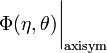$~\Phi(\eta,\theta)\biggr|_\mathrm{axisym}$$~=$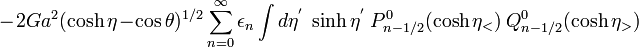$~ - 2Ga^2 (\cosh\eta - \cos\theta)^{1 / 2} \sum\limits^\infty_{n=0} \epsilon_n \int d\eta^' ~\sinh\eta^'~P^0_{n-1 / 2}(\cosh\eta_<) ~Q^0_{n-1 / 2}(\cosh\eta_>)$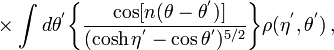$~ \times \int d\theta^' \biggl\{ \frac{ ~\cos[n(\theta - \theta^')]}{(\cosh\eta^' - \cos\theta^')^{5/2}} \biggr\} \rho(\eta^',\theta^') \, ,$ Wong (1973), p. 293, Eq. (2.55)

where,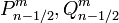$~P^m_{n-1 / 2}, Q^m_{n-1 / 2}$ are Associated Legendre Functions of the first and second kind of order,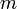$~m$, and half-integer degree,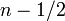$~n - 1/2$ (toroidal functions). Recognizing that the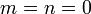$~m=n=0$ toroidal function of the second kind can be expressed in terms of the complete elliptic integral of the first kind via the relation,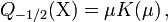$~Q_{-1 / 2}(\Chi) = \mu K(\mu)\, ,$     where,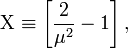$~\Chi \equiv \biggl[ \frac{2}{\mu^2} - 1\biggr] \, ,$

Abramowitz & Stegun (1995), p. 337, eq. (8.13.3)

we see that Version 3 of our integral expression for the Gravitational Potential of an Axisymmetric Mass Distribution may be rewritten as,$~\Phi(\eta,\theta)\biggr|_\mathrm{axisym}$$~=$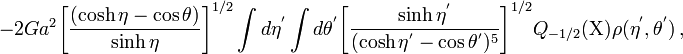$~ - 2Ga^2 \biggl[ \frac{(\cosh\eta - \cos\theta)}{\sinh\eta } \biggr]^{1 / 2} \int d\eta^' \int d\theta^' \biggl[ \frac{\sinh\eta^'}{(\cosh\eta^' - \cos\theta^')^5} \biggr]^{1 / 2} Q_{- 1 / 2}(\Chi) \rho(\eta^',\theta^') \, ,$ Cohl & Tohline (1999), p. 88, Eqs. (31) & (32a)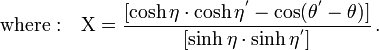$\mathrm{where:}~~~\Chi = \frac{[\cosh\eta \cdot \cosh\eta^' - \cos(\theta^' - \theta)]}{[\sinh\eta \cdot \sinh\eta^']} \, .$

Even though these two expressions for the axisymmetric potential are written entirely in terms of identically defined toroidal coordinate systems, they appear to be very different from one another. In the accompanying chapter titled, Using Toroidal Coordinates to Determine the Gravitational Potential, we detail step-by-step how the first of these expressions can be transformed into the second, thereby proving that they are indeed perfectly equivalent integral expressions for the gravitational potential of axisymmetric systems.

------------------------------

Wong (1973) actually derived a toroidal-coordinate-based integral expression for the Coulomb (equivalently, gravitational) potential that is applicable to all charge (mass) distributions, irrespective of geometric symmetries. The expression highlighted here — that is relevant to axisymmetric mass distributions — is a special case of this more general expression.